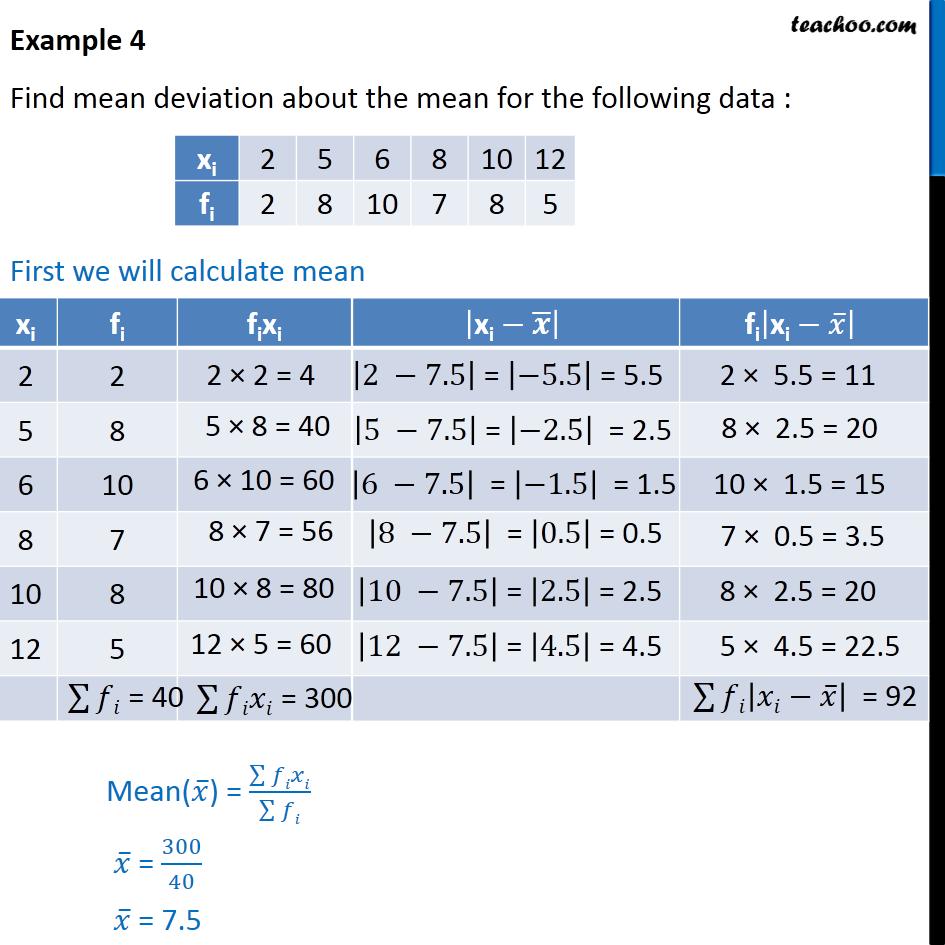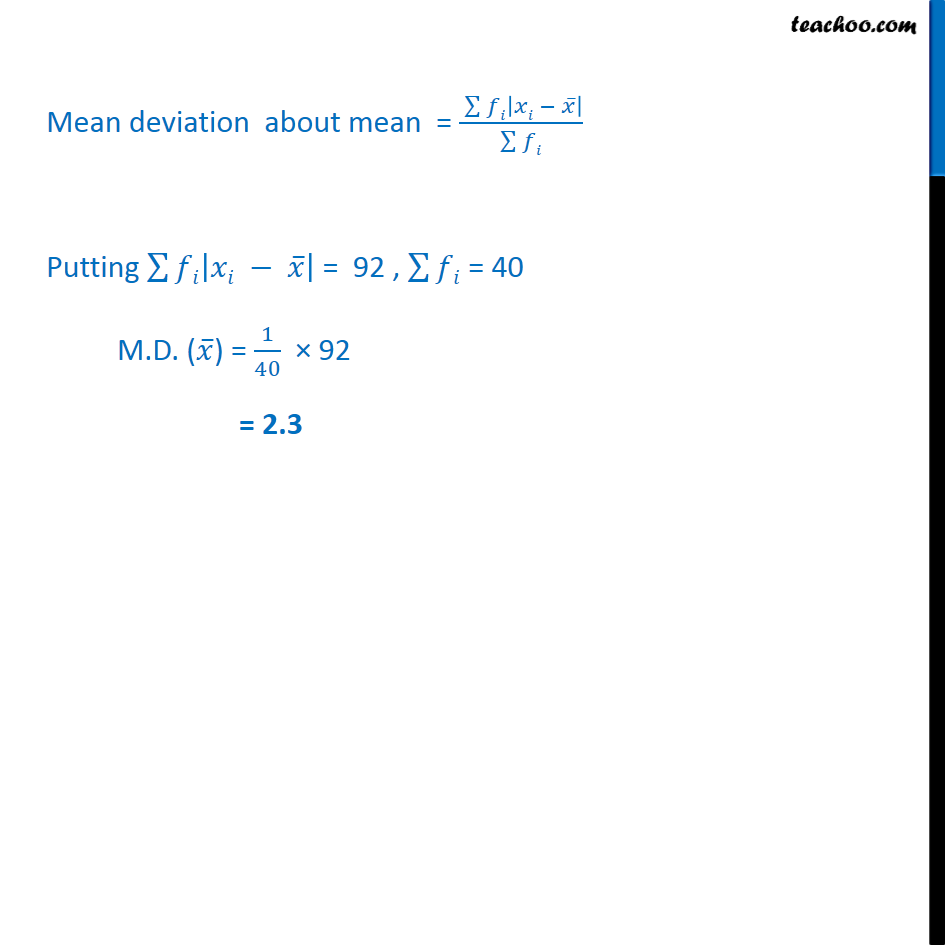Examples

Chapter 13 Class 11 Statistics
Serial order wiseLearn in your speed, with individual attention - Teachoo Maths 1-on-1 Class

### Transcript

Example 4 Find mean deviation about the mean for the following data : First we will calculate mean Mean deviation about mean = ﷮﷮𝑓𝑖 𝑥𝑖 − 𝑥﷯﷯﷯﷮ ﷮﷮𝑓𝑖﷯﷯ Putting ﷮﷮𝑓𝑖 𝑥𝑖 − 𝑥﷯﷯﷯ = 92 , ﷮﷮𝑓𝑖﷯ = 40 M.D. ( 𝑥﷯) = 1﷮40﷯ × 92 = 2.3